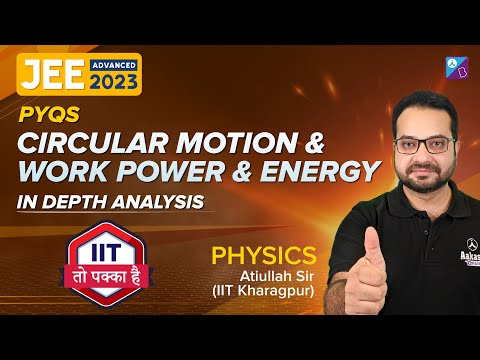# Unit of Power

Power, in general, is defined as the rate at which energy is transferred or converted or the rate of doing work. Thus, power can be calculated using the formula P = W / t. On the other hand, power is a scalar quantity and is basically the amount of energy consumed per unit of time which has no direction. While we have already talked about power in our other article, on this page students will find all the information regarding the unit of power.

## SI Unit of Power

According to popular standards, the SI unit of power is none other than the watt which is represented by the symbol (W). One joule per second is also equal to one watt. Interestingly, the name was kept in recognition of James Watt, who invented the condenser for the steam engine. He also coined the term horsepower, which is the older unit of power. It can be depicted as;

 1 watt = 1 J/s = 1 kg-m2/s3

### Other Power Units

Some of the common power units include ergs per second (erg/s), foot-pounds per minute, dBm, food calories per hour or kilocalories per hour, horsepower (hp), BTU per hour (BTU/h).

#### Units Of Power Conversions

 Units Abbreviation Equivalent Watt Unit Horsepower HP 746 watts Kilowatts kW 1×103W Megawatts MW 1×106W Gigawatts GW 1×109W decibel-milliwatts dBm 30 dBm = 1 W British Thermal Unit/Hour BTU/hr 3.412142 BTU/hr = 1 w Calories per Second cal/sec 0.24 calories per second cal/sec = 1 W

## The video explains the fundamentals of the concept of Power## Frequently Asked Questions – FAQs

Q1

### What is power?

Power is defined as the rate at which energy is transferred or converted or the rate of doing work.
Q2

### What is the formula to calculate power?

Power is given by the formula:
P = W / t
Q3

### What is the SI unit of power?

SI unit of power is the watt (W).
Q4

True.
Q5

### Name three power units.

Units of power are ergs per second (erg/s), horsepower (hp), and foot-pounds per minute.